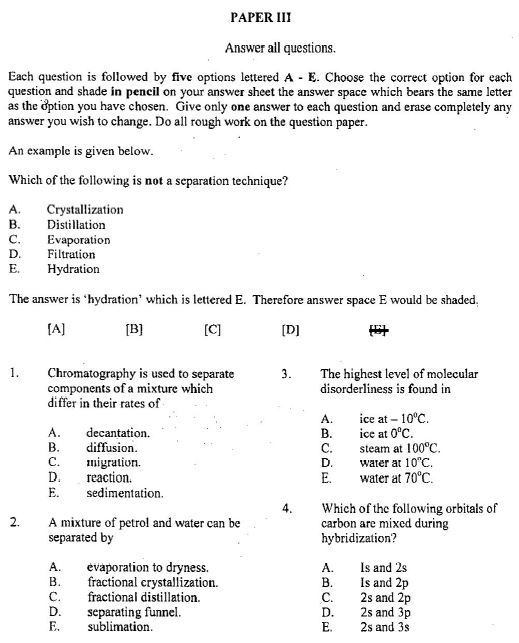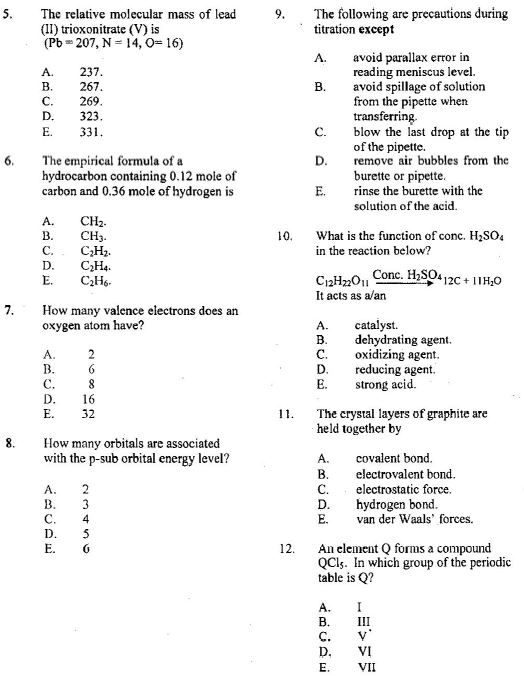# Expo: NECO GCE Chemistry Questions and Answers 2021/2022

Looking for free NECO GCE chemistry objective and theory questions and answers? You are in the right place. Here, I will be posting the correct exam answers here for all the candidates who will participate in the examination.

I will also post the past, likely and sample questions and answers for you to read and see how the exams will look like. All you have to do is to keep following my guidelines here.

This Neco gce chemistry exam is for Papers III & II: Objective & Essay and will take a total of 3hrs to write.

## How do I get the real NECO GCE chemistry questions and answers 2021?

The real questions and answers will be posted here for all students will participate in the SSCE (external) examination. The expo will be posted here 2 hours before the exam commences. So, save this page and visit later for your answers. You can also subscribe to our latest posts by tapping on the notification button below to enable you get answers on your phone once we post it here.

NEW NECO GCE TIMETABLE

New NECO timetable for ss3

NECO GCE physical education questions and answers

## Today’s NECO GCE chemistry Essay questions and answers

1. (a) State
(i) Pauli’s Exclusion principle;
(ii) Hund’s rule of maximum multiplicity.

(b) (i) Write the electronic configuration of each of the following ions of copper:
I.  Cu+;
II.  Cu2+. [29Cu]
(ii) Give the number of unpaired electrons in each of the ions in 1(b) (i) above.
(iii) State the type of reaction represented by the following equation: 2Cu+(aq)  →   Cu2+(aq) + Cu(s)
(iv) Write the formula of one compound of Cu+.

(c) (i) Name the type of radiation that will
II. be stopped by thin paper.
(ii) Give the charge on each of the radiations mentioned in 1(c) (i) above.
(iii) What term is used to describe each of the following nuclear processes?

I. Combination of two lighter nuclei to form a heavy nucleus.
II. Splitting of a heavy nucleus into two or more lighter nuclei.
III. Time required for one-half of the atoms of a radioactive  substance to decay.

(d) Arrange the following ions in order of increasing size.  Give a reason for your answer in each case.
I.   Li+, K+ , Na+;
II.  O2-,  F-,  N3-
(e) Determine the percentage composition of phosphorus and oxygen in phosphorus (V) oxide.
[P  =  31, O  =  16]

2. (a) (i) Define in terms of electron transfer
I.  oxidizing agent;
II.  reducing agent.
(ii) Write a balanced equation to show that carbon is a reducing agent.
(iii) State the change in oxidation number of the specie that reacted with carbon in 2 (a)(ii).

(b) A gas X has a vapour density of 32.  It reacts with sodium hydroxide solution to form salt and water only. It decolourizes acidified potassium tetraoxomanganate (VII) solution and reacts with H2S to form sulphur.  Using the information provided:
I. identify gas X;
II. state two properties exhibited by X;
III. give two uses of X.

(c) Consider the following substances: (i) sodium; (ii) lead (II) iodide; (iii) hydrogen; (iv) magnesium; (v) oxygen.
Which of the substances
(i) conducts electricity?
(ii) is produced at the cathode during electrolysis of H2SO4(aq)?
(iii) corresponds to the molecular formula AB2  ?
(iv) is an alkaline earth metal?

(d) (i) Define the term salt.
(ii) Mention two types of salt.
(iii) Give an example of each of the salts mentioned in 2(d)(ii) above.

(e) In a neutralization reaction, dilute tetraoxosulphate (VI) acid completely reacted with sodium hydroxide solution.
(i) Write a balanced equation for the reaction.
(ii) How many moles of sodium hydroxide would be required for the complete neutralization of 0.50 moles of  tetraoxosulphate (VI) acid?

3. (a) Consider the following reaction sequence.
(i) What process leads to the formation of K?
(ii) Write the formula of K.
(iii) Write the structural formula of L and name L.
(iv) Name An.
(v) Write the structure of M and name M.

(b) (i) What are carbohydrates?
(ii) Give one example each of a;
I. monosaccharide;
II. disaccharide;
III. polysaccharide.
(c)  A hydrocarbon Z with molecular mass 78 on combustion gave 3.385 g of CO2 and 0.692 g of H2O.  Determine the molecular formula of Z.
[H = 1, C=12, O=16]

4. (a) (i) Define covalent bond.
(ii)  Give two properties of covalent compounds
(iii) With the aid of a diagram, show how ammonia molecule is formed.
(iv) Illustrate with a diagram the formation of ammonium ion.
(v) What type of bond(s) exist(s) in
ammonia,
ammonium ion? [ 1H,  7 N]
(b) (i) Write three subatomic particles with their corresponding relative masses.
(ii) Name the possible states in which water can exist.
(c) (i) State Graham’s law of diffusion.
(ii) Arrange the following gases, He, CH4 and N2 in order of increasing rates of diffusion.  Give a reason for the order.
[H=1, He=4, C=12, N=14 ]

(d) Draw the structures of the following compounds:
(i) 2, 3-dimethylbutane;
(ii) 1, 4-dibromocyclohexane.

5. a) Write a balanced chemical equation for the reaction of butane gas with oxygen gas to form carbon dioxide and water vapor.
b) How many liters of oxygen are required to produce 2.0 liters of CO2?
c) How many liters of CO2 are produced from 11.6 g of butane at STP?
d) How many molecules of water vapor are produced from 5.6 liters of butane gas at STP?

6. (a) (i) What are acidic oxides?
(ii) Give one example of each of the following oxides:
I. acidic oxide;
II. basic oxide;
(III) amphoteric oxide;
(III) neutral oxide.

(b) (i) Define each of the following terms:
I. Heat of combustion;
II. Heat of neutralization.
(ii) Write an equation to illustrate each of the terms in 6(b)(i) above.
(iii) Given that the standard heat of combustion of butane (C4H10) is + 5877 kJmol-1, calculate the heat of combustion of 14.5 g butane.
[H  =  1, C  =  12]
(c) (i) Name two allotropes of sulphur.
(ii) State one difference between the two allotropes.
(d) (i) Give two characteristics of noble gases.
(ii) State one use each of
(I) He;
(II) Ar.
(e)  State what is observed on warming ammonium trioxonitrate (V) with sodiumhydroxide.

7. (a) (i) Define hard water.
(ii) Name two substances responsible for hardness in water.
(iii) Give two methods for the removal of hardness in water.
(b) (i) What are the raw materials required for the manufacture of
tetraoxosulphate (VI) acid by the contact process?
(ii) Write an equation for the reaction that requires a catalyst in the contact process.
(iii) State the catalyst used in 7(b)(ii).
(c) (i) Give two uses of sodium tetraoxosulphate (VI)
Consider the reaction represented by the following equation:
exposure to air Na2SO4 (aq) IOH2O (s) →  Na2SO4    H2O  + 9H2O (g)
(ii) What name is given to this type of reaction?
(iii) Calculate the solubility of Na2CO3 at 25oC, if 30.0cm3 of its saturated
solution at that temperature gave 1.80 g of the anhydrous salt.
[C=12, O=16, Na=23]

(d) (i) Define the term activated complex.
(ii) State one reason why a collision may not produce a chemical reaction.
(iii) The formation of water gas is represented by the following equation.
C(s) +  H2 O(g) →  CO (g) H2 (g)  ΔH = + 131   kJmol-1

(e) Draw an energy profile diagram for the reaction showing the
I.  activated complex
II.  enthalpy of reactants.

8. (a) (i) How many liters of methane are there in 8.00 grams at STP?
(ii) Calculate the density of carbon dioxide at 546 K and 4.00 atmospheres pressure.
(iii) What volume of O2 at 710. mm Hg pressure and 36°C is required to react with 6.52 g of CuS?
CuS(s) + 2 O2(g)   ®    CuSO4(s)

(b) (i) What is the molar mass

of a gas if 7.00 grams occupy 6.20 liters at 29°C and 760. mm Hg pressure?
(ii) At a particular temperature and pressure, 15.0 g of CO2 occupy 7.16 liters.  What is the volume of 12.0 g of CH4 at the same temperature and pressure?

(c) To prepare a sample of hydrogen gas, a student reacts 7.78 grams of zinc with acid:
Zn(s)   +   2 H+ (aq)    ®    Zn2+(aq)   +   H2(g)
The hydrogen is collected over water at 22°C and the total pressure of gas collected is 750. mm Hg. What is the partial pressure of H2?  What volume of wet hydrogen gas is collected?

NOTE: That there is nothing like Neco expo chemistry online. Do not be deceived by fraudsters posing with fake NECO answers on the internet.

## NECO GCE chemistry obj questions and answers

#### NECO GCE Chemistry Objectives.## Area of concentration for the examination

Gases:

• Kinetic-molecular theory
• pressure
• barometer, manometer
• temperature
• absolute zero temperature
• relationship between pressure, volume, temperature
• Boyle’s Law
• Charles’ Law
• Ideal Gas Law R = 0.08206  L atm/mol K
• molar volume
• STP
• molar volume @ STP = 22.4 L
• molar mass and density of a gas
• gas stoichiometry
• partial pressure.